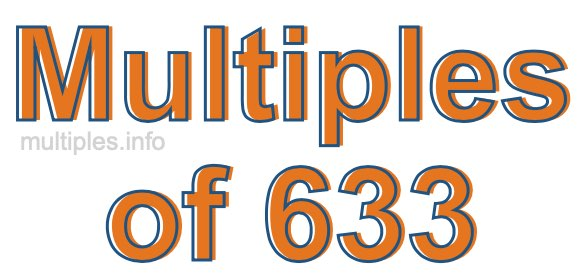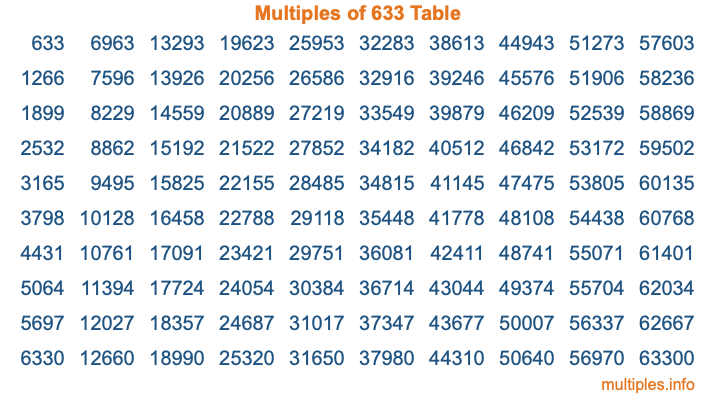Multiples of 633Welcome to the Multiples of 633 page. Here we will first teach you everything you will ever need to know about the multiples of 633, and then give you a study guide summary of everything we taught you to make sure you remember it all. Use this page to look up facts and learn information about the multiples of 633. This page will make you a multiples of six hundred thirty-three expert!

Definition of Multiples of 633
Multiples of 633 are all the numbers that when divided by 633 equal an integer. Each of the multiples of 633 are called a multiple. A multiple of 633 is created by multiplying 633 by an integer.

Therefore, to create a list of multiples of 633, you start with 1 multiplied by 633, then 2 multiplied by 633, then 3 multiplied by 633, and so on for as long as you want. Thus, the list of the first five multiples of 633 is 633, 1266, 1899, 2532, and 3165. To see a larger list of multiples of 633, see the printable image of Multiples of 633 further down on this page. We also have a category where you can choose any nth multiple of 633.

Multiples of 633 Checker
The Multiples of 633 Checker below checks to see if any number of your choice is a multiple of 633. In other words, it checks to see if there is any number (integer) that when multiplied by 633 will equal your number. To do that, we divide your number by 633. If the the quotient is an integer, then your number is a multiple of 633.

Is  a multiple of 633?

Least Common Multiple of 633 and ...
A Least Common Multiple (LCM) is the lowest multiple that two or more numbers have in common. This is also called the smallest common multiple or lowest common multiple and is useful to know when you are adding our subtracting fractions. Enter one or more numbers below (633 is already entered) to find the LCM.

Check out our LCM Calculator if you need more details about the Least Common Multiple or if you need the LCM for different numbers for adding and subtraction fractions.

nth Multiple of 633
As we stated above, 633 is the first multiple of 633, 1266 is the second multiple of 633, 1899 is the third multiple of 633, and so on. Enter a number below to find the nth multiple of 633.

th multiple of 633

Multiples of 633 vs Factors of 633
633 is a multiple of 633 and a factor of 633, but that is where the similarities end. All postive multiples of 633 are 633 or greater than 633. All positive factors of 633 are 633 or less than 633.

Below is the beginning list of multiples of 633 and the factors of 633 so you can compare:

Multiples of 633: 633, 1266, 1899, 2532, 3165, etc.

Factors of 633: 1, 3, 211, 633

As you can see, the multiples of 633 are all the numbers that you can divide by 633 to get a whole number. The factors of 633, on the other hand, are all the whole numbers that you can multiply by another whole number to get 633.

It's also interesting to note that if a number (x) is a factor of 633, then 633 will also be a multiple of that number (x).

Multiples of 633 vs Divisors of 633
The divisors of 633 are all the integers that 633 can be divided by evenly. Below is a list of the divisors of 633.

Divisors of 633: 1, 3, 211, 633

The interesting thing to note here is that if you take any multiple of 633 and divide it by a divisor of 633, you will see that the quotient is an integer.

Multiples of 633 Table
Below is an image of the first 100 multiples of 633 in a table. The table is in chronological order, column by column. The first column has the first ten multiples of 633, the second column has the next ten multiples of 633, and so on.The Multiples of 633 Table is also referred to as the 633 Times Table or Times Table of 633. You are welcome to print out our table for your studies.

Negative Multiples of 633
Although not often discussed or needed in math, it is worth mentioning that you can make a list of negative multiples of 633 by multiplying 633 by -1, then by -2, then by -3, and so on, to get the following list of negative multiples of 633:

-633, -1266, -1899, -2532, -3165, etc.

Multiples of 633 Summary
Below is a summary of important Multiples of 633 facts that we have discussed on this page. To retain the knowledge on this page, we recommend that you read through the summary and explain to yourself or a study partner why they hold true.

There are an infinite number of multiples of 633.

A multiple of 633 divided by 633 will equal a whole number.

633 divided by a factor of 633 equals a divisor of 633.

The nth multiple of 633 is n times 633.

The largest factor of 633 is equal to the first positive multiple of 633.

633 is a multiple of every factor of 633.

633 is a multiple of 633.

A multiple of 633 divided by a divisor of 633 equals an integer.

633 divided by a divisor of 633 equals a factor of 633.

Any integer times 633 will equal a multiple of 633.

Multiples of a Number
Here you can get the multiples of another number, all with the same attention to detail as we did for multiples of 633 on this page.

Multiples of
Multiples of 634
Did you find our page about multiples of six hundred thirty-three educational? Do you want more knowledge? Check out the multiples of the next number on our list!